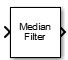Documentation

# Median Filter

Median filter

• Library:
• DSP System Toolbox / Filtering / Filter Designs

DSP System Toolbox / Statistics## Description

The Median Filter block computes the moving median of the input signal along each channel independently over time. The block uses the sliding window method to compute the moving median. In this method, a window of specified length moves over each channel sample by sample, and the block computes the median of the data in the window. This block performs median filtering on the input data over time. For more details, see Algorithms.

## Ports

### Input

expand all

Data over which the block computes moving median. The block accepts real-valued or complex-valued multichannel inputs, that is, m-by-n size inputs, where m ≥ 1, and n ≥ 1. The block also accepts variable-size inputs. During simulation, you can change the size of each input channel. However, the number of channels cannot change.

Data Types: `single` | `double`
Complex Number Support: Yes

### Output

expand all

The size of the moving median output matches the size of the input. The block uses the sliding window method to compute the moving median. For more details, see Algorithms.

Data Types: `single` | `double`
Complex Number Support: Yes

## Parameters

expand all

Window length specifies the length of the sliding window.

• `Code generation`

Simulate model using generated C code. The first time you run a simulation, Simulink® generates C code for the block. The C code is reused for subsequent simulations, as long as the model does not change. This option requires additional startup time but provides faster simulation speed than ```Interpreted execution```.

• `Interpreted execution`

Simulate model using the MATLAB®  interpreter. This option shortens startup time but has slower simulation speed than ```Code generation```.

## Block Characteristics

 Data Types `double` | `single` Multidimensional Signals `No` Variable-Size Signals `Yes`

expand all

Watch now